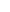當前位置：東奧會計在線 >  免費試聽 > ACCA

Depreciation

IAS 16 allows two methods of depreciation:

u Straight line

Depreciation = (cost –residual value)/Useful economic life

Or

(cost – residual value) * % (depreciated rate)

u Reducing balance (Depreciated on Net book value)

Depreciation = NBV @ opening * % (depreciated rate)

u Additional factors to consider

l Depreciation starts when the asset is ready for its intended use and not from when it starts to be used, until the asset is derecognized , even if it is idle.

l The useful life of assets and depreciation method should be reviewed at least at each financial year end, and when necessary, revised. (IAS 8)

l Separate component of PPE should be capitalized as separate assets and depreciated over their useful lives

l No depreciation for land

5816人已學習